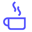a concise shot of dev knowledge
Become a Contributor
Concise shots of dev knowledge

RELATED TAGS

two strings
isomorphic
algorithm

# How to check if two strings are isomorphicEdpresso Team

Two strings are isomorphic if one-to-one mapping is possible for every character of the first string to every character of the second string.

For example, consider the two strings: “ACAB” and “XCXY”. We can map the characters of the first string to the characters of the second string as follows:

• ‘A’ maps to ‘X’.
• ‘C’ maps to ‘C’.
• ‘B’ maps to ‘Y’.

Hence, the two strings are isomorphic.

Now, consider the strings “AAB” and “XYZ”. These are not isomorphic since ‘A’ cannot have a mapping to both ‘X’ and ‘Y’.

Another way to look at it is that two strings are isomorphic if each character in the first string can be replaced by a character to get the second string, and vice-versa.

## Algorithm

A naive solution would be to check if every character in the first string is mapped to the same character in the second string. However, another pass would still need to be made in the reverse direction to check if every character in the second string is mapped to the same character in the first string. This solution has a time complexity of $O(n^{2})$.

An optimal solution uses hashing to solve the problem in $O(n)$. Consider the following illustration that outlines the algorithm:

1 of 7

## Code

#include <iostream>
#include <map>
#include <set>

using namespace std;

bool isIsomorphic(string str1, string str2)
{
// Two strings cannot be isomorphic if they have different lengths.
if (str1.length() != str2.length())
return false;

// Use C++'s built in map to store mappings of str1 chars to str2 chars.
map<char, char> map;

// Use a set to keep track of already mapped characters.
set<char> set;

for (int i = 0; i < str1.length(); i++)
{
char c1 = str1[i], c2 = str2[i];

// If c1 has been encountered before:
if (map.find(c1) != map.end())
{
// Return false if first occurrence of c1 is mapped to
// a different character.
if (map[c1] != c2)
return false;
}

// If c1 is encountered for the first time, it has not been mapped yet:
else
{
// Return false if c2 is already mapped to some other char in str1
if (set.find(c2) != set.end())
return false;

// All checks passed. So insert in the map, and the set.
map[c1] = c2;
set.insert(c2);
}
}
return true;
}

// Driver code
int main()
{
string str1 = "ACAB";
string str2 = "XCXY";

if (isIsomorphic(str1, str2))
cout << str1 << " and " << str2 << " are isomorphic.";
else
cout << str1 << " and " << str2 << " are not isomorphic.";
}

RELATED TAGS

two strings
isomorphic
algorithm
RELATED COURSES

View all Courses

Keep Exploring

Learn in-demand tech skills in half the time